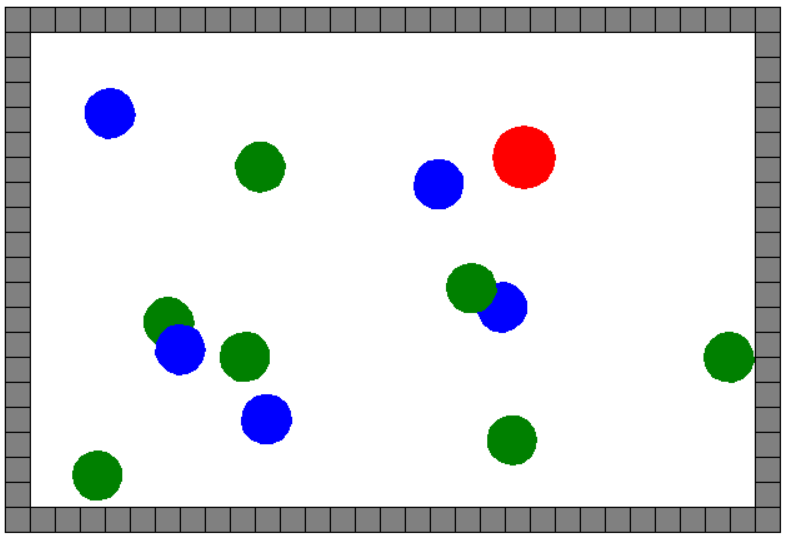﻿ Python turtle编写简单的球类小游戏_python_脚本之家
python# Python turtle编写简单的球类小游戏

turtle （小海龟） 是 Python 内置的一个绘图模块，其实它不仅可以用来绘图，还可以制作简单的小游戏。本文将利用Turtle制作一个简单的球类小游戏，感兴趣的可以学习一下

## 1. 前言

turtle （小海龟） 是 Python 内置的一个绘图模块，其实它不仅可以用来绘图，还可以制作简单的小游戏，甚至可以当成简易的 GUI 模块，编写简单的 GUI程序。

turtle 模块的使用相对而言较简单，对于基础方法不做讲解。只聊 turtle 模块中稍难或大家忽视的地方。

## 2. 需求描述## 3. 制作流程

### 3.1 初始化变量

```import turtle
import random
import math
'''

'''
# 游戏区域的宽度
game_wid = 600
# 游戏区域的高度
game_hei = 400
# 砖块的大小，以及每一个小球初始大小
cell = 20
# 红球初始大小
red_size = cell
# 红色小球
red_ball = None
# 存储绿色小球的列表
green_balls = []
# 存储蓝色小球的列表
blue_balls = []
# 红色小球的方向 当前方向 0 向右，90 向上 180 向左 -90 向下
dir = 0
```

### 3.2 通用函数

```'''

'''
def rand_pos():
# 水平有 30 个单元格，垂直有 20 个单元格
x = random.randint(-14, 14)
y = random.randint(-9, 9)
return x * cell, y * cell
```

```'''

'''
def draw_square(color):
if color is not None:
# 的颜色就填充
turtle.fillcolor(color)
turtle.begin_fill()
for i in range(4):
turtle.fd(cell)
turtle.left(90)
if color is not None:
turtle.end_fill()
```

```'''

name:画笔名称
color:可选项
'''
def custom_shape(name, size):
turtle.begin_poly()
turtle.penup()
turtle.circle(size)
turtle.end_poly()
cs = turtle.get_poly()
turtle.register_shape(name, cs)
```

`turtle.register_shape(name, shape)` 方法参数说明：

• name： 自定义形状的名称。
• shape： 由开发者绘制的形状。

`cs = turtle.get_poly()` 可以理解为获取到刚绘制的图形，然后使用 `turtle.register_shape(name, cs)` 注册画笔形状，以后就可以随时使用此形状。

```'''

'''
def move_pos(pen, pos):
pen.penup()
pen.goto(pos)
pen.pendown()

```

• `pen` : 画笔对象。
• `pos`：要移到的目标地。

`turtle` 模块提供有很多事件，可以以交互式的方式使用`turtle``turtle` 模块中主要有 2 类事件：键盘事件、点击事件。因 `turtle` 的工作重点还是绘制静态图案上，其动画绘制比较弱，所以它的事件少而简单。

```'''

'''
def dir_right():
global dir
dir = 0
def dir_left():
global dir
dir = 180
def dir_up():
global dir
dir = 90
def dir_down():
global dir
dir = -90

'''

'''
def register_event():
for key, f in {"Up": dir_up, "Down": dir_down, "Left": dir_left, "Right": dir_right}.items():
turtle.onkey(f, key)
turtle.listen()
'''

'''
def is_meet_qt():
global dir
if red_ball.xcor() < -220:
dir = 0
if red_ball.xcor() > 240:
dir = 180
if red_ball.ycor() > 140:
dir = -90
if red_ball.ycor() < -120:
dir = 90

```

### 3.3 游戏角色函数

Tips： 墙体由主画笔绘制。

```'''

'''
def draw_blocks():
# 隐藏画笔
turtle.hideturtle()
# 上下各30个单元格，左右各 20 个单元格
for j in [30, 20, 30, 20]:
for i in range(j):
# 调用前面绘制正方形的函数
draw_square('gray')
turtle.fd(cell)
turtle.right(90)
turtle.fd(-cell)
# 回到原点
move_pos(turtle, (0, 0))
```

```def init_ball(pos, color, shape):
#  由主画笔创建新画笔
ball = turtle.Turtle()
ball.color(color)
# 指定新画笔的形状，如果不指定，则为默认形状
ball.shape(shape)
# 移到随机位置
move_pos(ball, pos)
# 移动过程要不显示任何轨迹
ball.penup()
return ball
```

• `pos`： 创建画笔后画笔移动的位置。
• `color`：指定画笔和填充颜色。
• `shape`： 已经定义好的画笔形状名称。

```def ran_gb_ball(balls, color):
# 随机创建蓝色、绿色小球的频率，
# 也就是说，不是调用此函数就一定会创建小球，概率大概是调用 5 次其中会有一次创建
ran = random.randint(1, 5)
# 随机一个角度
a = random.randint(0, 360)
# 1/5 的概率
if ran == 5:
turtle.tracer(False)
# 每一个小球就是一只画笔
ball = init_ball(rand_pos(), color, 'ball')
ball.seth(a)
# 添加到列表中
balls.append(ball)
turtle.tracer(True)
```

`turtle.tracer(False)` 方法的作用：是否显示画笔绘制过程中的动画。`False` 关闭动画效果，`True` 打开动画效果。

```def gb_ball_m(balls):
s = 20
a = random.randint(0, 360)
r = random.randint(0, 10)
for b in balls:
b.fd(s)
if b.xcor() < -220 or b.xcor() > 240 or b.ycor() > 140 or b.ycor() < -120:
b.goto(rand_pos())
```

```'''

'''
def r_g_b_meet():
global red_size
# 红色小球的坐标
s_x, s_y = red_ball.pos()
# 迭代绿色小球，蓝色小球列表
for bs in [green_balls, blue_balls]:
for b in bs:
# 计算蓝色或绿色小球坐标
f_x, f_y = b.pos()
# 计算和红色球之间的距离
x_ = math.fabs(s_x - f_x)
y_ = math.fabs(s_y - f_y)
# 碰撞距离：两个球的半径之和
h = cell + red_size
if 0 <= x_ <= h and y_ >= 0 and y_ <= h:
if b in green_balls:
# 遇到绿色球红球变大
red_size += 2
if b in blue_balls:
# 遇到蓝色球红球变大
red_size -= 2
# 关键代码
custom_shape('red', red_size)
return True
return False
```

### 3.4 让小球动起来

```def ball_move():
red_ball.seth(dir)
red_ball.fd(40)
# 检查红球是否碰到墙体
is_meet_qt()
# 随机创建绿色小球
ran_gb_ball(green_balls, 'green')
# 随机创建蓝色小球
ran_gb_ball(blue_balls, 'blue')
# 让绿色小球移动
gb_ball_m(green_balls)
# 让蓝色小球移动
gb_ball_m(blue_balls)
# 检查红球是否碰到蓝色、绿色小球
r_g_b_meet()
# 定时器
turtle.ontimer(ball_move, 100)
```

```if __name__ == "__main__":
# 关闭动画效果
turtle.tracer(False)
# 注册事件
register_event()
# 定制 2 种画笔形状
for name in ['red', 'ball']:
custom_shape(name, cell)
# 主画笔移动墙体的左上角
move_pos(turtle, (-300, 200))
# 绘制墙体
draw_blocks()
red_ball = init_ball(rand_pos(), 'red', 'red')
turtle.tracer(True)
# 让红球移动起来
ball_move()
#
turtle.done()
```